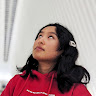Postulate is the best way to take and share notes for classes, research, and other learning.

# quantum shmantumLaura Gao
Feb 22, 2022Last updated Feb 22, 20225 min read

Quantum mechanics is like my DELF B2 French proficiency exam. Right now, its outcome is a hazy cloud, a combination of the two possible results of "pass" and "fail." As we mere humans cannot predict the future, I am unable know the result until Test Day comes on March 10th at which point it's etched into the unalterable fabric of the past, just like like I cannot know if a photon is horizontally or vertically polarized until it passes through a polarizing filter, at which point I can never revert it to the superposition it was before. It's as if when I write the exam, the superposition of it's outcome will collapse into a single basis state of

|pass\rangle $|pass\rangle$
or
|fail\rangle $|fail\rangle$
, unable to go back to the ignorant bliss (like I would want if it measures
|fail\rangle $|fail\rangle$
), just we can never revert a collapsed superposition (as information about the its complex amplitudes and phase have been lost).

Because of these similarities, it almost won't be innaccurate to extend this analogy by writing the outcome of my exam as

\alpha|pass\rangle + \sqrt{1-\alpha} |fail\rangle $\alpha|pass\rangle + \sqrt{1-\alpha} |fail\rangle$
. By studying more, I can increase the value of
\alpha $\alpha$
, shift more of the probability from
|pass\rangle $|pass\rangle$
to
|fail\rangle $|fail\rangle$
, just like how quantum computers can alter the wavefunction of a qubit by shooting radio waves at very specific frequencies at them. But no matter how hard I try, I can never bring
\alpha $\alpha$
to
1 $1$
, but only closer and closer as I approach an asymptote, just like how quantum computers will never return a basis state with 100% certainty due to noise and decoherence (interference from other objects altering the superposition undesirably, which is a huge pain in the butt for quantum computing researchers). Because
\alpha $\alpha$
can never be
1 $1$
, there will always be some randomness in my exam results. We can use this to answer the long-running hard work vs luck debate. We can say that the magnitude of
\alpha $\alpha$
is like the "hard work" portion of success -- the part that we can control. But whether the wavefunction ends up collapsing as pass or fail, like whether an electron in superposition collapses to spin up or spin down, is completely up to the dice of God. This is why sometimes, I place 2nd in Canada at FBLA, a business competition, despite studying for a total of 2 hours the night before -- because no matter how small
\alpha $\alpha$
is, there's still room for you to get really really lucky. Hard work plays a big component of success, just like the probability amplitudes of a superposition largely determine what state it will collapse to, but luck also exerts its influence, like how the superposition ends up collapsing falls at the whim of randomness.

In this way, one could say that every point in the future is like a superposition, just like every subatomic particle in the map of the physical world is one. In either case, these superpositions do not exist separately. Let me explain. One of these such points is whether I get accepted into EPFL, a university in the French-speaking region of Switzerland. Since the B2 certification is a hard requirement for the application, the wavefunction of these two entangled superposition exists as

\alpha|Pass\_B2 \otimes Accepted\_EPFL\rangle + \sqrt{1=\alpha} |Fail\_B2 \otimes Rejected\_EPFL \rangle $\alpha|Pass\_B2 \otimes Accepted\_EPFL\rangle + \sqrt{1=\alpha} |Fail\_B2 \otimes Rejected\_EPFL \rangle$
. I will never get accepted into EPFL while failing the B2, just Schrodinger will never discover the entangled state of his cat (a faulty example of superposition but nevertheless a decent intuitive explanation of entanglement)
\dfrac{|atom\_not\_decayed \otimes cat\_alive\rangle + |atom\_decayed \otimes cat\_dead\rangle}{\sqrt{2}} $\dfrac{|atom\_not\_decayed \otimes cat\_alive\rangle + |atom\_decayed \otimes cat\_dead\rangle}{\sqrt{2}}$
as
|atom\_decayed \otimes cat\_alive\rangle $|atom\_decayed \otimes cat\_alive\rangle$
. For a more accurate example, a quantum circuit in the common entangled state of
\dfrac{|00\rangle+|11\rangle}{\sqrt{2}} $\dfrac{|00\rangle+|11\rangle}{\sqrt{2}}$
(known as a bell state) will measure to either
|00\rangle $|00\rangle$
or
|11\rangle $|11\rangle$
, but never
|01\rangle $|01\rangle$
or
|10\rangle $|10\rangle$
. Both future events and superpositions share the commonality that the outcome of different unknowns can affect each other.

Like a superposition, you only get to measure each exam once. Unlike most superpositions, we can not recreate most exams to measure them multiple times. No matter how good quantum computers get, we can never completely eliminate noise and decoherence, even in the post-quantum-supremacy era. This is why for every qubit, there might be two "error-correcting" qubits so that quantum computers can be sure that their result is correct. No quantum hardware engineer will be so cocky about their creation as to say it will return a correct result if you only run your program once, on a single set of qubits. Hence, one should not put their faith into the outcome of a single exam to reflect their skill as a person, just like we will never rely on the measurement of a single superposition. Just like it is only through the distribution of the measurement outcomes of a superposition that a scientist can have a good understanding of what the wavefunction looked like, it is only through the overall distribution of passes and fails you accumulate throughout many months that one can get a good understanding of their performance in a course.

As for my B2 exam, however, I only have one shot. I'll just have to study hard tonight to maximize the magnitude of the amplitude of my

|pass\rangle $|pass\rangle$
vector.

Originally written for my SCH3U course taught by Ms. Woods